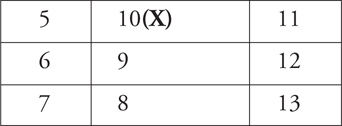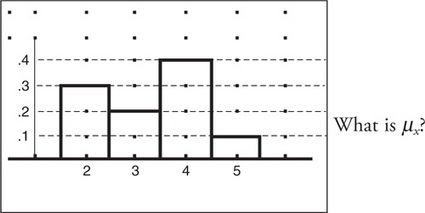# AP Statistics Practice Test 11

### Test Information10 questions23 minutes

1. What are the mean and standard deviation of a binomial experiment that occurs with probability of success 0.76 and is repeated 150 times?

2. Which of the following is the primary difference between an experiment and an observational study?

3. The regression analysis of question 20 indicated that "R-sq = 98.1%." Which of the following is (are) true?

I. There is a strong positive linear relationship between the explanatory and response variables.

II. There is a strong negative linear relationship between the explanatory and response variables.

III. About 98% of the variation in the response variable can be explained by the regression on the explanatory variable.

4. A hypothesis test is set up so that P(rejecting H0when H0is true) = 0.05 and P(failing to reject H0when H0is false) = 0.26. What is the power of the test?

5. For the following observations collected while doing a chi-square test for independence between the two variables Aand B, find the expected value of the cell marked with "X."6. The following is a probability histogram for a discrete random variable X.7. A psychologist believes that positive rewards for proper behavior are more effective than punishment for bad behavior in promoting good behavior in children. A scale of "proper behavior" is developed. μ1= the "proper behavior" rating for children receiving positive rewards, and μ2= the "proper behavior" rating for children receiving punishment. If H0: μ1μ2= 0, which of the following is the proper statement of HA?

8. Estrella wants to become a paramedic and takes a screening exam. Scores on the exam have been approximately normally distributed over the years it has been given. The exam is normed with a mean of 80 and a standard deviation of 9. Only those who score in the top 15% on the test are invited back for further evaluation. Estrella received a 90 on the test. What was her percentile rank on the test, and did she qualify for further evaluation?

9. Which of the following statements is (are) true?

I. In order to use a χ2procedure, the expected value for each cell of a one- or two-way table must be at least 5.

II. In order to use χ2procedures, you must have at least 2 degrees of freedom.

III. In a 4 × 2 two-way table, the number of degrees of freedom is 3.

10. When the point (15,2) is included, the slope of regression line (y= a+ bx) is b= -0.54. The correlation is r= -0.82. When the point is removed, the new slope is -1.04 and the new correlation coefficient is -0.95. What name is given to a point whose removal has this kind of effect on statistical calculations?